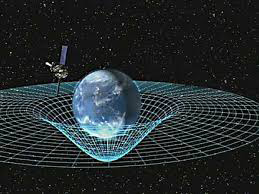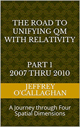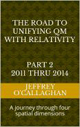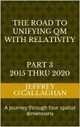# The dynamics of space-time

One question that has yet to be answer regarding Einstein relativistic theories is how time and space interact to create the past, present and future.

Einstein side step this question by assuming, as he put it “there exists in this four-dimensional structure [space-time] no longer any sections which represent “now” objectively, the concepts of happening and becoming are indeed not completely suspended, but yet complicated. It appears Therefore, more natural to think of physical reality as a four-dimensional existence, instead of, as hitherto, the evolution of a three-dimensional existence.”Marina Cort’s, a cosmologist from the Royal Observatory, Edinburgh defined what has come to be called the block universe to help us understand how Einstein may have viewed the past present and future.

Basically, she asks us to imagine a regular chunk of cement. It has three dimensions but we live in four dimensions: the three spatial dimensions plus one time dimension. A block universe is a four-dimensional block, but instead of being made of cement, it is made of space-time. And all of the space and time of the Universe are there in that block.”

We can’t see this block, we’re not aware of it, as we live inside the cement of space-time. And we don’t know how big the block universe we live in is: “We don’t know if space is infinite or not. Or time – we don’t know whether it has a beginning or if it will have an end in the future. So, we don’t know if it’s a finite chunk of space-time or an infinite chunk.”

In other words, the past, present, and future exist simultaneously and are locked in a non-dynamic, unchanging block of space-time with the rigidity of cement.

However, to understand why Einstein he had to make this assumption one must first define what space and time are.

For example, some define time only in the abstract saying that is an invention of the human consciousness that gives us a sense of order, a before and after so to speak.Â  To physicist’s it is a measure of the relative interval between events which is measured in units of time such as seconds.

While space can be defined as the arena where those events occur.  We use the measurements of inch or meter to define the position of those event in that arena.

The problem Einstein with defining how energy causes a dynamic change in a space-time environment that define “happening and becoming” or the future, may have been due to the fact that he mathematically defined it in terms of a melding of time with space which have different units. Therefore, because, in mathematics if the dimensions or units on the left and right-hand sides don’t agree the equation are nonsense it is hard to imagine how the future is created in terms of space-time. This is why he said “It appears Therefore, more natural to think of physical reality as a four-dimensional existence, instead of, as hitherto, the evolution of a three-dimensional existence.

However, the fact he found that definition unsatisfactory is evident when he said that “concepts (or causality) of happening and becoming are indeed not completely suspended, but yet complicated” indicate that he was aware of this.

In other words, Einstein realized that causality of the future in terms of a dynamic process was something that must be considered.

Yet, Einstein gave us an alternative way of understanding “happening and becoming” when he defined the relationship between energy and space-time in terms of the constant velocity of light and the equation E=mc^2 because in doing so he provided a method of converting a unit of time and energy in a space-time environment to its equivalent unit of space in four *spatial* dimensions.  Additionally, because the velocity of light is constant, he also defined a one to one quantitative and qualitative correspondence between his space-time universe and one made up of only four *spatial* dimensions.

In other words, Einstein’s mathematics actually defined two mathematically equivalent physical models of the universe one consisting of four-dimensional space-time and one of only four *spatial* dimensions.

In his space-time model he mathematically defined all forms of energy including gravity and the kinetic energy of motion in terms of a curvature or displacement in the “surface” of four-dimensional space-time manifold. However, in his equivalent model consisting of only four *spatial” dimensions he would have defined them in terms of a displacement or curvature in the “surface” of a three-dimensional space manifold with respect to a fourth *spatial* dimension.

As was mentioned earlier, it was evident Einstein realized the difficulty of deriving the future or happenings in terms of his space-time model when he said “no longer any sections which represent “now” objectively, the concepts of happening and becoming are indeed not completely suspended, but yet complicated.

However, the same is not true of the equivalent model mentioned above consisting of only four *spatial* dimensions because defining the causality of change of the future in those terms would eliminate the problem mentioned above with the incompatibility of space and time.

Yet, before we can define the future in terms of the dynamics four *spatial* dimension we must first explain how it interacts with time to cause it to dilate and shorten length of objects when it is in relative motion with respect to an observer.

For example, one can show as was mentioned earlier by using the Einstein mathematics the kinetic energy of motion can be understood in terms of a displacement in a “surface” of a three-dimensional space manifold with respect to a fourth spatial dimension as well one in four-dimensional space-time.

One can understand how this would effect time and the length of objects in relative motion by assuming the perspective of two “2 dimensional creatures are living on the surface of two pieces of paper resting on a desktop.

Also, assume the two creatures can view the surfaces of the other piece of paper, which are separated a pencil.

If the diameter of the pencil is increased, the curvature between the surfaces of the two pieces of paper will increase.

Each of these creatures, when viewing the other piece of paper will only perceive the two-dimensional translation of the three-dimensional curvature generated by the pencil.

Therefore, each will view the distance between two points on the surface of the other as shorter since they will view that distance as a two-dimensional translation of the three-dimensional curvature in the surface of the paper.

Similarly, because three-dimensional beings could only “view” a three-dimensional translation of a “curvature” or displacement in four *spatial* dimension caused by the motion of a reference frame they will measure distance or length in them as being longer than they would be if viewed as an observer who is not in relative motion to it.

The “movement” of time on both surfaces will also be affected.

Each of the two-dimensional creatures mentioned earlier will view the others time as moving slower because the three-dimensional curvature in the paper makes the distance between events longer than the two-dimensional translation of those events.  Therefore, it will take longer for events “move” through a curvature in three-dimensional space relative to the time it would take for them to move through two-dimensional translation on the others surface caused by that curvature.

Similarly, time will become dilated in reference frames that are in motion because the curvature generated on its three-dimensional “surface” caused by its relative motion will result the distance between events to be longer than it with respect to the distances measured in reference frames observe on them assumed them to be stationary.  Therefore, they will view time in a reference frame that is in motion relative to them as moving slower than if they were in that reference frame.

As show above both of these models, the one based on the physical existence of four dimensions space-time and the existence of only four spatial dimensions make identical predictions as to the relativistic properties of space and time, therefore which one you chose to define the physical structure of our universe must be based, in part on how you view the future.

However, Einstein space-time interpretation did not allow him to define the dynamic changes in our environment that we call the future because he mathematically defined them in terms of a melding of time with space which have different units.  Therefore, he had to assume that the past present and future was locked in a block of cement.

However, as was shown above the same is not true if one interprets his equation in terms of four *spatial* dimensions because all they all have the same units.

Yet, because as was mentioned earlier both of these models are mathematically equivalent and since we cannot physically observe either a time or a fourth *spatial* dimension, we must look to the affects they would have on the ones we can observe or in this case how we perceive the future to determine which one of these physical models is correct.

In other words, if you view it as something that dictates the past and present you will probably chose his space-time model.  However, if you view the future as a dynamic interaction of the past with the present you will most likely choose the model based on only four *spatial* dimensions.

Latter Jeff

 The Road to Unifying QM with Relativity part 1 2007 thru 2010Ebook \$8.00Paper Back \$15.00 The Road to Unifying QM with Relativity part 2 2011 thru 2014Ebook \$8.00Paper Back \$16.00 The Road to Unifying QM with Relativity part 3 2015 thru 2020Ebook \$8.00Paper Back \$18.00